## The point (-6, 4) lies on a straight line that has a gradient of -2. What is the equation of this line?

Question

The point (-6, 4) lies on a straight line that has a gradient of -2. What is the
equation of this line?

in progress 0
2 months 2021-07-22T22:13:35+00:00 1 Answers 5 views 0

1. Given:

Point on straight line = (-6,4)

To find:

The equation of the line.

Solution:

Point-slope form: If a line passes through the point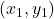with slope m, then the equation of the line is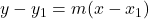The line passes through the point (-6,4) with slope -2. So, the equation of the line is: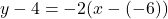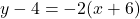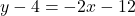Adding 4 on both sides, we get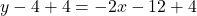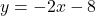Therefore, the equation of the line is.